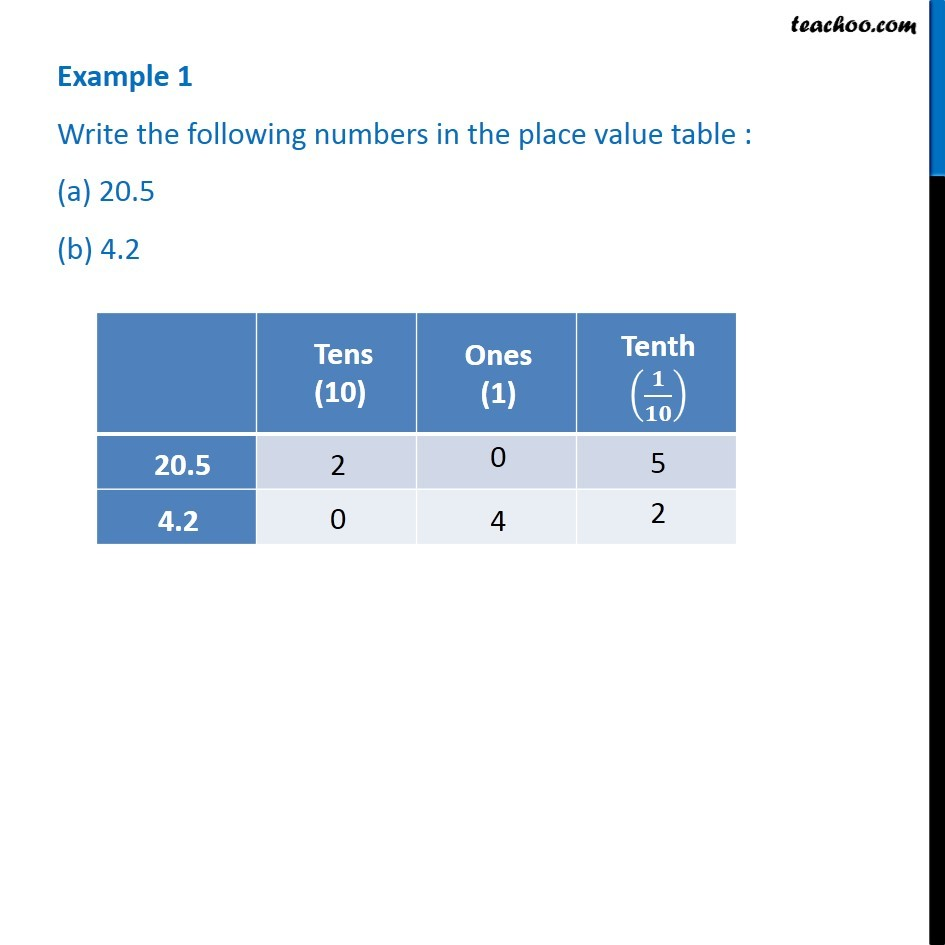Examples

Chapter 8 Class 6 Decimals
Serial order wiseLearn in your speed, with individual attention - Teachoo Maths 1-on-1 Class

### Transcript

Question 1 - Chapter 8 Class 6 - NCERT Solution Write the following numbers in the place value table : (a) 20.5 (b) 4.2 For 20.5 Tens (10) = 2 Ones (1) = 0 Tenths (1/10) = 5 For 4.2 Tens (10) = 0 Ones (1) = 4 Tenths (1/10) = 2